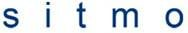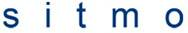# Homework check on Harmonic Motion

roldy
1.
A mass of 0.5 kg hangs on a spring. The stiffness of the spring is 100 N/m, and the mechanical resistance is 1.4 kg/s. The force (N) driving the system is f=2cost5t.

(a) What will be the steady-state values of the displacement amplitude, speed amplitude, and average power dissipation?

(b) What is the phase angle between speed and force?

(c) What is the resonance frequency and what would be the displacement amplitude, speed amplitude, and average power dissipation at this frequency and for the same force magnitude as in (a)?

(d) What is the Q of the system, and over what range of frequencies will the power loss be at least 50% of its resonance value?

2.
eq. 1eq. 2eq. 3
Zm2=Rm2 + (omega*m-s/omega)^2

## The Attempt at a Solution

m= 0.5 kg
stiffness=k=s(in my book)=100 N/m
mechanical resistance=c=Rm(in my book)=1.4 kg/s
f=2cost5t

(a) I used eq. 1 and found the displacement amplitude to be .023 m or 2.3 cm.

To get the speed amplitude, I differentiated the displacement equation. The only thing I differentiated was the forcing function since that is the only thing that's dependent on time. So basically I multiplied the derivative of f by .023. This resulted in -.115 m/s. So the velocity amplitude is .115 m/s or 11.5 cm/s.

For the average power dissipation I used eq. 2
And using eq. 3 I found what Zm was.

power dissipation=.009 W

(b) Here I'm not so sure I'm right. I said that it was pi/2. F=ma, and the acceleration response is a cosine function (differentiated the velocity response from (a)). The speed response is a sine function. Cosine and sine are out of phase by pi/2 radians. Does my logic make sense? Or is there something I messed up.

(c) Here I'm also not sure I did this right. In my book it says the following:

The resonance angular frequency omega_o is defined as that at which the mechanical reactance X_m vanishes and the mechanical impedance is pure real with its minimum value, Z_m=R_m.

So in order for Z_m to equal R_m in eq. 3, the term in (...) must be zero.

omega*m-s/omega=0
omega*m=s/omega
omega^2=s/m
omega=(s/m)^1/2

I got 14.142 rad/s. This does not seem right because it is more than the natural frequency of the driving force which is 5 rad/s. Using this number in the displacement amplitude I came up with .101 m or 10.1 cm. This made me question my result for the resonance frequency.

(d) I didn't bother doing yet because of the questionable answer I got above.

Any help would be greatly appreciated. I've spent the past 2 days trying to figure this out.

Last edited by a moderator:

## Answers and Replies

Staff Emeritus
Homework Helper
FYI, please don't boldface entire posts in the future.## The Attempt at a Solution

m= 0.5 kg
stiffness=k=s(in my book)=100 N/m
mechanical resistance=c=Rm(in my book)=1.4 kg/s
f=2cost5t

(a) I used eq. 1 and found the displacement amplitude to be .023 m or 2.3 cm.
Looks reasonable.
To get the speed amplitude, I differentiated the displacement equation. The only thing I differentiated was the forcing function since that is the only thing that's dependent on time. So basically I multiplied the derivative of f by .023. This resulted in -.115 m/s. So the velocity amplitude is .115 m/s or 11.5 cm/s.
Agreed. vampl is ω·xampl.

For the average power dissipation I used eq. 2
And using eq. 3 I found what Zm was.

power dissipation=.009 W
I'll trust you on that one, it has been a while since I studied this stuff myself.
(b) Here I'm not so sure I'm right. I said that it was pi/2. F=ma,
No, not quite. Remember that "F=ma" really means
F = ma
The driving force is just one force in the sum (there is also the spring and the damping forces). They want the phase angle between v and Fdrive, not ∑F.

Does your book or class lecture notes not give an equation for x(t), given a sinusoidal Fdrive(t)?

... and the acceleration response is a cosine function (differentiated the velocity response from (a)). The speed response is a sine function. Cosine and sine are out of phase by pi/2 radians. Does my logic make sense? Or is there something I messed up.

(c) Here I'm also not sure I did this right. In my book it says the following:

The resonance angular frequency omega_o is defined as that at which the mechanical reactance X_m vanishes and the mechanical impedance is pure real with its minimum value, Z_m=R_m.

So in order for Z_m to equal R_m in eq. 3, the term in (...) must be zero.

omega*m-s/omega=0
omega*m=s/omega
omega^2=s/m
omega=(s/m)^1/2

I got 14.142 rad/s. This does not seem right because it is more than the natural frequency of the driving force which is 5 rad/s. Using this number in the displacement amplitude I came up with .101 m or 10.1 cm. This made me question my result for the resonance frequency.
That looks reasonable to me. There is no reason why the driving frequency has to be close to the resonance frequency. Also makes sense because, for driving well below resonance, you should have xampl≈Fampl/k, which is approximately true here.
(d) I didn't bother doing yet because of the questionable answer I got above.

Any help would be greatly appreciated. I've spent the past 2 days trying to figure this out.

roldy
The only equation that I came across for x(t) is the following:

x=[F/(omega*Zm)]sin(omega*t-Theta)

I also found this:

The actual speed is:

u=(F/Zm)cos(omega*t-Theta)

I could use this but I don't have a value for t.

Staff Emeritus
Homework Helper
That seems odd that your book doesn't have a complete derivation. Does it tell you what Zm is, but doesn't say what θ is?

One way to calculate θ is to use your equation for x, and plug it into the full differential equation that describes the system. So, all force terms (driving, spring, and damping) added together must equal mx''. Then it's a lot of messy trig and algebra to figure out what θ is.

IIRC, the algebra may be easier to work with if you use as a trial solution
x=Xampl·eiωt-θ

roldy
This book is horrible. Zm=Rm+jXm where Xm=omega*m-s/omega.

$$\Theta$$ is the phase angle.

Also, $$\Theta$$=tan-1(Xm/Rm)

I'll give it another shot and see if I can find something.